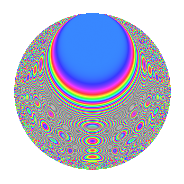# Properties

 Label 430.2.pLevel 430 Weight 2 Character orbit p Rep. character $$\chi_{430}(59,\cdot)$$ Character field $$\Q(\zeta_{14})$$ Dimension 132 Newform subspaces 1 Sturm bound 132 Trace bound 0

# Related objects

## Defining parameters

 Level: $$N$$ = $$430 = 2 \cdot 5 \cdot 43$$ Weight: $$k$$ = $$2$$ Character orbit: $$[\chi]$$ = 430.p (of order $$14$$ and degree $$6$$) Character conductor: $$\operatorname{cond}(\chi)$$ = $$215$$ Character field: $$\Q(\zeta_{14})$$ Newform subspaces: $$1$$ Sturm bound: $$132$$ Trace bound: $$0$$

## Dimensions

The following table gives the dimensions of various subspaces of $$M_{2}(430, [\chi])$$.

Total New Old
Modular forms 420 132 288
Cusp forms 372 132 240
Eisenstein series 48 0 48

## Trace form

 $$132q + 22q^{4} - 4q^{5} - 4q^{6} + 12q^{9} + O(q^{10})$$ $$132q + 22q^{4} - 4q^{5} - 4q^{6} + 12q^{9} + 8q^{11} - 10q^{14} - 20q^{15} - 22q^{16} - 4q^{19} + 4q^{20} - 24q^{21} + 4q^{24} - 16q^{25} + 12q^{26} + 40q^{29} + 24q^{31} - 4q^{35} + 128q^{36} - 56q^{39} - 28q^{41} - 8q^{44} - 80q^{45} - 12q^{46} - 136q^{49} - 56q^{50} + 172q^{51} + 16q^{54} - 4q^{56} + 16q^{59} - 8q^{60} - 44q^{61} + 22q^{64} + 42q^{65} + 8q^{66} - 62q^{69} + 12q^{70} - 8q^{71} - 4q^{74} - 122q^{75} - 52q^{76} - 64q^{79} - 4q^{80} - 56q^{81} + 24q^{84} - 72q^{85} - 10q^{86} + 16q^{89} + 2q^{90} - 24q^{91} - 20q^{94} + 106q^{95} - 4q^{96} - 104q^{99} + O(q^{100})$$

## Decomposition of $$S_{2}^{\mathrm{new}}(430, [\chi])$$ into newform subspaces

Label Dim. $$A$$ Field CM Traces $q$-expansion
$$a_2$$ $$a_3$$ $$a_5$$ $$a_7$$
430.2.p.a $$132$$ $$3.434$$ None $$0$$ $$0$$ $$-4$$ $$0$$

## Decomposition of $$S_{2}^{\mathrm{old}}(430, [\chi])$$ into lower level spaces

$$S_{2}^{\mathrm{old}}(430, [\chi]) \cong$$ $$S_{2}^{\mathrm{new}}(215, [\chi])$$$$^{\oplus 2}$$

## Hecke Characteristic Polynomials

There are no characteristic polynomials of Hecke operators in the database